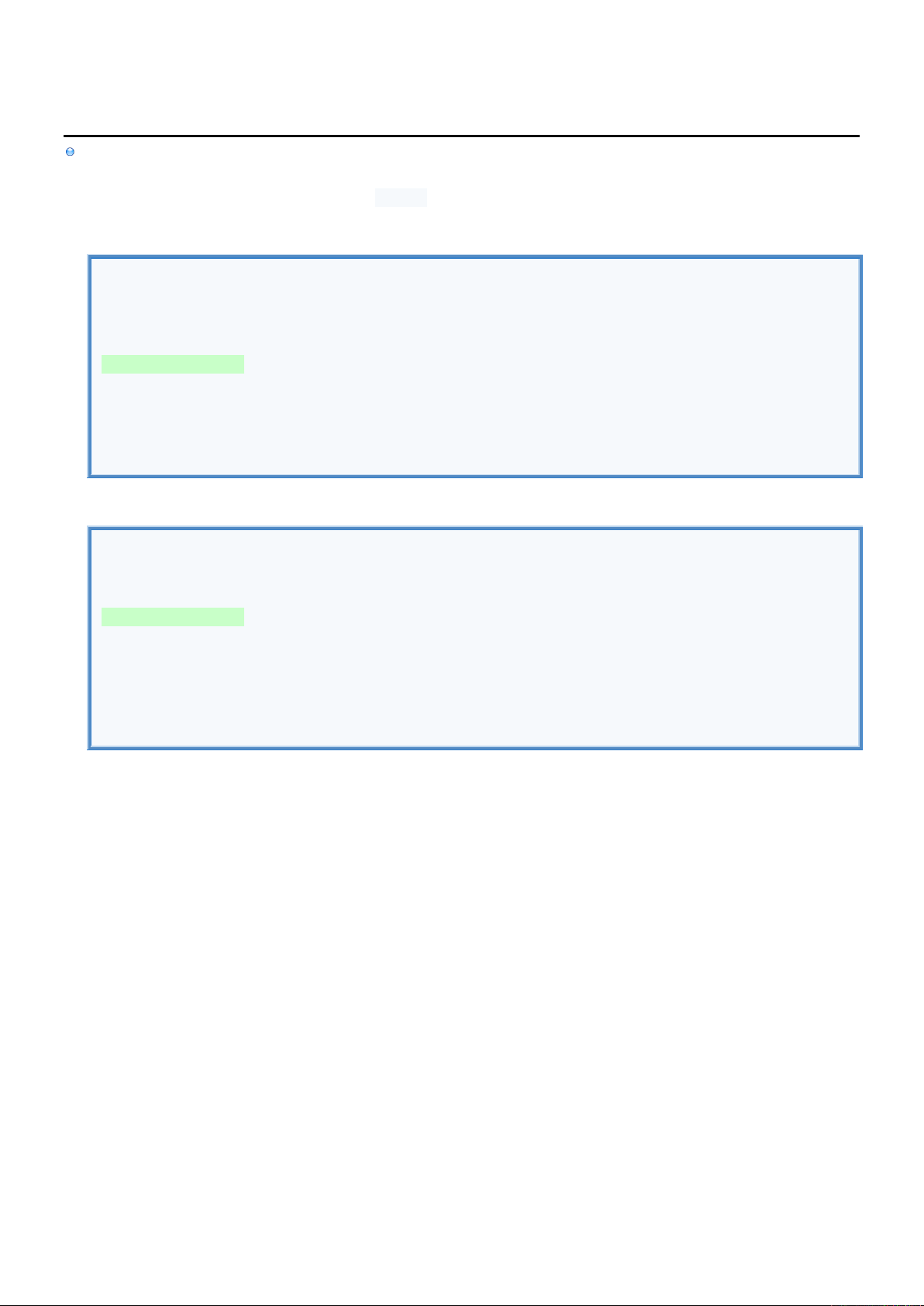2
2
.
.
3
3
.
.
3
3
S
S
t
t
a
a
t
t
e
e
m
m
e
e
n
n
t
t
s
s
-
-
C
C
o
o
n
n
d
d
i
i
t
t
i
i
o
o
n
n
a
a
l
l
I
I
n
n
f
f
o
o
Conditional Statements execute its Body single time if Condition is TRUE
if ... else Statement executes single section for which condition is TRUE
when Statement is used instead if ... else Statement when all conditions evaluate the same variable
if ... else
//TEST VARIABLES.
var number = 10
var text = "Hello"
//IF ELSE STATEMENT (CAN RETURN VALUE).
var result : String =
if (number == 10 ) "Number is 10" //Can ommit {} for single line
else if (text == "Hello") { "Text equals Hello" } //Don't use return to return value
else if (text == "World") { "Text equals World" }
else { print("No match"); "No match found" }
print(result)
when [R]
//TEST VARIABLES.
var number = 10
//WHEN STATEMENT (CAN RETURN VALUE).
var result : String =
when(number) {
10 -> "number = 10" //Can ommit {} for single line
20, 30 -> { "number = 20 or 30" } //It doesn't fall through to subsequent case
else -> { print("No match"); " No match found" } //Don't use return to return value
}
print(result)